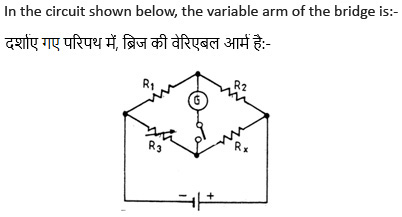﻿ All awareness for Bank PO | Clerk | Railway | IBPS PO | IBPS Clerk | SSC ﻿

### Engineering

ISBT Reasoning for all banking PO,Clerk,IBPS PO,Railway,SSC,IAS,OAS Exams

Previous1234Next

#####1) R1 2) R2 3) R3 4) R4 5) None of theseView Answer
##### if there are b branches and n nodes the number of equations will be
 1) n-1 2) b 3) b-n 4) b-n+1 5) None of theseView Answer
##### The minimum requirements for causing flow of current are
 1) a voltage source,a resistor, and a switch. 2) a voltage source and a conductor 3) a power source and a bulb 4) a voltage source,a conductor,an ammeter and a switch 5) None of these
Answer : a voltage source and a conductorView Answer
##### Due to the existence of  which of the following, the current flowing in an electrical circuit ?
 1) mmf 2) emf 3) Resistance 4) Reluctance 5) None of theseView Answer
##### In a linear system, an input of 5 sin wt produces an output of 10 cos wt. The output corresponding to input 10 cos wt will be equal to -
 1) + 5 sin wt 2) - 5 sin wt 3) + 20 sin wt 4) – 20 sin wt. 5) None of these
Answer : – 20 sin wt.
Explanation :

Sin wtCos wt Differentiating

Cos wt2 Sin wt

An input of 10 Cos wt will cause a

Response of – 20 Sin wt.View Answer
##### For an SCR, di/dt protection is achieved through the use of  which of the following series ?
 1) R in series with SCR 2) L in series with SCR 3) RL in series with SCR 4) RLC in series with SCR 5) None of these
Answer : L in series with SCR
Explanation : For an SCR, di/dt protection is achieved through the used of L in series with SCR. A snubber circuit connected across an SCR is to suppress dv / dt.View Answer
##### The electric field lines and equipotential lines -
 1) Are parallel to each other 2) Are one and the same 3) Cut each other orthogonally 4) Can be inclined to each other at any angle 5) None of these
Answer : Cut each other orthogonally
Explanation : Since no electric can exist along any surface, all points of which are at the same potential, electric field lines and equipotential lines are orthogonal to each other.View Answer
##### The resistance of a strip of copper of rectangular cross-section is 2 ohm. A metal of resistivity twice that of copper is coated on its upper surface to a thickness equal to that of copper strip.  The resistance of composite strip will be - ( DRDO Exam. 2013)
 1) 6 ohms 2) 4/3 ohms 3) 3/2 ohms 4) 3/4 ohms 5) None of these
Answer : 4/3 ohms
Explanation : Copper and coated metal strips have resistance of 2 ohms and 4 ohms respectively.
These two strips are in parallel. Hence the resistance of the composite strip will be (2x4) / (2+4) = 4/3 ohms.View Answer
##### What is the current rating of MCB, when it is used for 165 litre refrigirators ?
 1) 2 amps 2) 4 amps 3) 1.5 amps 4) 7.5 amps 5) None of these
Answer : 2 ampsView Answer
##### A  network consists of linear resistors and ideal voltage source. If the value of the resistors are doubled, then voltage across each resistor is -
 1) halved 2) doubled 3) increases four lines 4) not changed 5) None of these
Answer : not changedView Answer

Previous1234Next